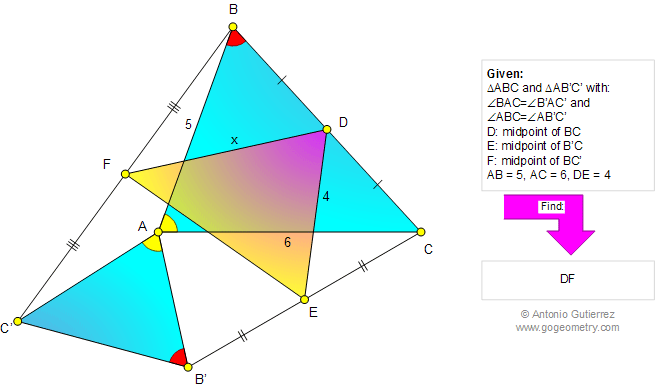# Online Geometry Problem 772: Similar triangles with a Common Vertex, Equal Angles, Midpoints, Metric relations. Level: High School, College, Mathematics Education

 The figure below shows triangles ABC and AB'C' with the common vertex A, angle BAC = angle B'AC', and angle ABC=AB'C'. Points D, E, F are the midpoints of BC, B'C, and BC', respectively. If AB = 5, AC = 6, and DE = 4, find DF.Home | Search | Geometry | Problems | All Problems | Open Problems | Visual Index | 771-780 | Triangles | Midpoints | Angles | Similarity | Email | Solution / comment | By Antonio Gutierrez